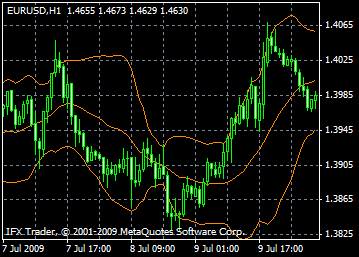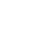## Instant account opening

Get a letter of instructions#### For mobile devices

InstaForex Bonuses
Make a deposit
Money withdrawal

# Bollinger bands indicator formula and settings: বিবরণ, সমন্বয় এবং অ্যাপ্লিকেশন

The Bollinger Bands indicator is a technical analysis instrument reflecting current fluctuations of an asset price (e.g. a share, commodity or currency).

The Bollinger Bands are calculated based on a standard deviation of a moving average. It is usually depicted on the price dynamics chart.

The Bollinger Bands are similar to the Envelopes. The difference between these two indicators is that borders of the Envelopes are situated above and below the moving average at a fixed distance, which is expressed in percent; while borders of the Bollinger Bands are built on the distance equal to the number of standard deviations. As the value of a standard deviation depends on volatility, the Bollinger Bands regulate their width on their own: it widens when the market is volatile; and it shrinks in stable periods.

The Bollinger Bands are usually plotted on a price chart, but can be applied to an indicator chart as well. Like the Envelopes, the Bollinger Bands are based on the idea that prices tend to hold within the upper and lower limits of the bands. The unique feature of the Bollinger Bands as an indicator is their variable width that is due to price volatility. During periods of high volatility, the bands widen, giving prices a space. During periods of low volatility, the Bollinger Bands narrow holding prices within their boundaries.

The key features of this indicator are:

1. Abrupt changes in prices after band narrowing that reflects decreasing volatility.

2. If prices go beyond band boundaries, the current trend is expected to continue.

3. If highs and lows beyond the bands are followed by highs and lows within the bands, a trend reversal is possible.

4. A price movement started from one of the band boundaries usually reaches the opposite boundary.

The latest observation is useful for forecasting price targets.#### Calculation

The Bollinger Bands are made up of three lines. The middle line (MIDDLE LINE, ML)  is a simple moving average.

ML = SUM [CLOSE, N]/N

The upper line (TOP LINE, TL) is the middle line moved upwards by a certain number of standard deviations (D).

TL = ML + (D*StdDev)

The lower line (BOTTOM LINE, BL) is the middle line moved downwards by the same number of standard deviations.

BL = ML – (D*StdDev)

Where:

SUM (..., N) – sum for N periods

CLOSE – closing price

N – the number of periods used for calculation

SMA – simple moving average

SQRT – square root

StdDev – standard deviation:

StdDev = SQRT(SUM[(CLOSE – SMA(CLOSE, N))^2, N]/N)

It is recommended to use 20-period simple moving average as the middle line and two standard deviations to determine band boundaries. Besides, moving averages of less than 10 periods are not very efficient.

﻿
বন্ধআমাদের বিশেষজ্ঞগণ
আপনাকে পাঁচ মিনিটের মধ্যে
কল করবে
ওয়েবসাইটের মাধ্যমে আমরা
আপনার কার্যক্রম পর্যালোচনা করব এবং
আপনার সকল প্রশ্নের জবাব দিব!
পছন্দের সংযোগের ধরণ
পছন্দের ভাষা
• English
• Русский
• العربية
• Bahasa Indonesia
• Bahasa Melayu
• বাংলা
• Български
• 中文
• Español
• हिन्दी
• Asụsụ Igbo
• Português
• اردو
• ไทย
• Українська
• Tiếng Việt
• Èdè Yorùbá
একটি কলব্যাক অনুরোধ গ্রহণ করা হয়েছে।
আমাদের বিশেষজ্ঞগণ যত দ্রুত সম্ভব আপনার সাথে যোগাযোগ করবে।
ভুল হয়েছে।
দয়াকরে আবার চেষ্টা করুন।
এখন কথা বলতে পারবেন না?
আপনার প্রশ্ন জিজ্ঞাসা করুন চ্যাট.